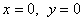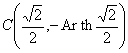3. 反双曲函数的定义、图形与特征

[反双曲函数的定义及其对数表达式]

 `函  数` `记  号` 对数表达式 反双曲正弦 若 x = sh y, 则 y = Ar sh x反双曲余弦 若 x = ch y, 则 y = Ar ch x反双曲正切 若 x = th y, 则 y = Ar th x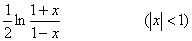反双曲余切 若 x = cth y, 则 y = Ar cth x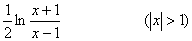反双曲正割 若 x = sech y, 则 y = Ar sech x反双曲余割 若 x = csch x, 则 y = Ar csch x`[反双曲函数的图形与特征]`
`   反双曲正弦曲线                     反双曲余弦曲线`
`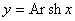`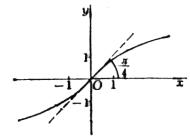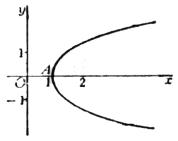`   曲线关于原点对称.                         曲线关于x轴对称.`
`   拐点（同曲线对称中心）：                  顶点：`
`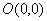，该点切线斜率为1`
`   反双曲正切曲线                                   反双曲余切曲线`
`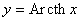``   曲线关于原点对称.                  曲线关于原点对称.`
`   拐点（同曲线对称中心）：            不连续点：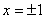`
`，该点切线斜率为1            渐近线：`
`   反双曲正割曲线                     反双曲余割曲线`
``曲线关于x轴对称.                             曲线关于原点对称.

顶点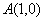不连续点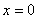拐点渐近线